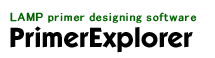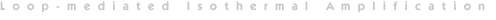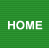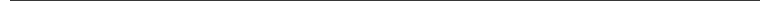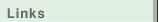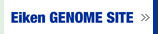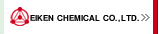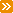Introduction (operating environment)Operation of each screen (designing regular primers/designing loop primers)AppendixA Guide to LAMP primer designing (Manual)：in preparation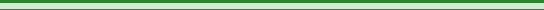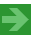3. Appendix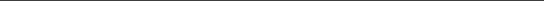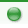Tm value calculation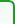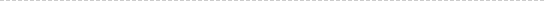The primer's Tm value is calculated from equation 1).

Tm = ΔH×1000/ (ΔS + R ln (C/ 4)) - 273.15 + 16.6 log [Na+] ............................ 1)
where
 R : Gas constant = 1.987 cal/ (mol-deg) ΔH : Enthalpy (kcal/mol) ΔS : Entropy (eu) C : Oligo-nucleotide concentration (M) [Na+] : Sodium ion concentration (M)* C/ 4 is employed when the target sequence is Non-self complementary. C/ 4 is replaced with C, when the target sequence is self-complementary. ** Concentration of magnesium ion is converted into that of sodium ion. [Na+]Mg = 4 [Mg2+]raw(1/2) [Na+]adjusted = [Na+]raw + [Na+]Mg ================================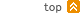Primer end's stability calculation3' or 5' end stability is calculated according to equation 2).

ΔG(total) = ΣΔG(i) + ΔG(Init.w/term.G-C) + ΔG(Init.w/term.A-T) + ΔG(sym) ............................ 2)

Based on Nearest-neighbor method, ΔG (free energy) of 6bp nucleotide at 3' or 5' end is calculated for the estimation of the end stability.
When the primer end 's ΔG is no more than -5kcal/ mol, it is defined to be stable according to the criterion for the end stability. This criterion is variable according to the target sequence and primer designing condition.
* Refer to reference (2) for the parameter value employed in ΔG calculation.
Refer to reference (3), (4) and (5) for the parameter value employed in ΔG calculation when the end strands are mismatched (for example A to G or C to A).
* Prerequisite condition
The target sequence ("N" nucleotides) is split into two nucleotide base pieces, and the nucleotide at the position "n" on the target sequence is defined as n (i) and its sequence as seq (i), where 1 ≤ n ≤ N. Therefore, seq (i) = n (i) + n (i+1), where 1 ≤ i ≤ N -1. The complementary sequence to seq (i) is defined as rev_seq (i). Free energy of these pieces is defined as ΔG (i) when they anneal to each other (whereas rev_seq (i) is not always complementary to seq (i)).

for example) GAACCTG → GA, AA, AC, CC, CT, TG
 Sequence seq (1) seq (2) seq (3) seq (4) seq (5) seq (6) GAACCTG GA AA AC CC CT TG(Parameter)
 ΔG(total) : Sum of the free energy ΔG(i) : Free energy of seq (i) ΔG(Init.w/term.G-C) : Initiation parameter when the nucleotide at 5' or 3' end is G or C ΔG(Init.w/term.A-T) : Initiation parameter when the nucleotide at 5' or 3' end is A or T ΔG(sym) : Symmetry adjustment. Adjustment value for the self-complementary sequence
 * Initiation parameter is employed to the calculation of both end stability. For example 1) 5' - AGGCTT - 3' ΔG(total) = ΣΔG(i) + 2ΔG(Init.w/term.A-T) For example 2) 5' - AGGCTG - 3' ΔG(total) = ΣΔG(i) + ΔG(Init.w/term.A-T) + ΔG(Init.w/term.G-C)References (1) John SantaLucia,Jr. et al. (1996) Biochemistry 35, 3555-3562 (2) John SantaLucia,Jr. (1998) Proc.Natl.Acad.Sci.USA 95, 1460-1465 (3) Hatim T.Allawi and John SantaLucia,Jr. (1998) Biochemistry 37, 2170-2179 (4) Hatim T.Allawi and John SantaLucia,Jr. (1998) Biochemistry 37, 9435-9444 (5) Nicolas Peyret et al. (1999) Biochemistry 38, 3468-3477Target rangePrimer Information filePrimer Information file Loop primers are used for the acceleration of LAMP reaction in addition to the regular LAMP primer (FIP, BIP, F3 and B3). For Loop primer designing, the information of not only the target sequence but the position of the FIP, BIP, F3 and B3 are required. This information is included in the Primer Information file, and is inputted into PrimerExplorer V3. Creation of Primer Information file Download the Primer information file from the Selected Primer Sets page.Introduction (operating environment)Operation of each screen (designing regular primers/designing loop primers)AppendixA Guide to LAMP primer designing (Manual)：in preparation